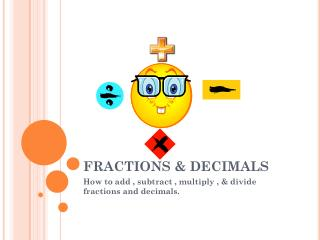DownloadDownload PresentationFRACTIONS & DECIMALS

FRACTIONS & DECIMALS

Télécharger la présentationFRACTIONS & DECIMALS

- - - - - - - - - - - - - - - - - - - - - - - - - - - E N D - - - - - - - - - - - - - - - - - - - - - - - - - - -
Presentation Transcript

1. FRACTIONS & DECIMALS How to add , subtract , multiply , & divide fractions and decimals.

2. FRACTIONS : ADDITION 1.Rewrite fractions with common denominators a. If you have a mixed #, rewrite the whole # in front of the “new” fraction with the common denominator. b. If you have a whole #, rewrite the whole number and write the fraction as 0 over the denominator of the fraction. 7/12 + 3/4 = 7/12 + 9/12 = 16/12=1 1/3 2. Denominators stay the same 3.Add numerators 4.Add whole #’s 5.Simplify a. Rewrite improper fractions as mixed numbers b. Divide by common factors to simplify fractions

3. FRACTIONS: SUBTRACTION 1.Rewrite fractions with common denominators a. If you have a mixed #, rewrite the whole # in front of the “new” fraction with the common denominator. b. If you have a whole #, rewrite the whole number and write the fraction as 0 over the denominator of the fraction. 2. Denominators stay the same 3/8 - 1/4 = 3/8 – 2/8 = 1/8

4. FRACTIONS: MULTIPLICATION • Change mixed numbers to improper fractions a. Multiply the whole number by the denominator b. Add that to the numerator 2 3/4 x 5/8= 2.Cancel out 11/4 x 5/8= 55/32 = 1 23/32 3. Multiply the numerator times numerator and the denominator times the denominator 4. Simplify a. Rewrite improper fractions as mixed numbers b. Divide by common factors to simplify fractions

5. FRACTIONS: DIVISION • Change improper fractions to mixed numbers 2. Rewrite first number 3.Change the division to multiplication 4.Flip the numbers of the second fractions 5. Cancel out if possible 6.Multiply numerators (top #s) 7. Multiply denominators (bottom 3s) 8. Simplify a. Rewrite improper fractions as mixed numbers b. Divide by common factors to simplify fractions 1/4 divided by 3/5 1/4 x 5/3 = 5/12

6. DECIMALS : ADDITION • Line up decimals • Add 2.5 + 8.0 10.5

7. DECIMALS: SUBTRACTION • Line up decimals • Be careful with borrowing • Subtract 10. -0.98 9.02

8. DECIMALS: MULTIPLICATION • Don’t have to line up decimals • Multiply as if there is no decimals • Count the # of #’s behind the decimals in the problem & put that many #’s behind the decimal in the answer 5.1 -1 x .13 -2 153 +510 .663

9. DECIMALS : DIVISION • Move decimal in divisor so that it is a whole # • Move the decimal in the dividend the same # of times • Divide .25 5.75 = 23

10. I GET IT!!!

11. By: Abby miller!!